# Types of Numbers

In engineering you’ll come across different types of numbers. For example in Control Engineering, Physics (AC Electric Machines) complex numbers are widely used. In Microcontrollers, Embedded Systems and Programming an integer number is a common term.

For this reason we are going to have a short introduction in the different Types of Numbers. This article will clear the path to a more detail article on complex numbers.

## Natural Numbers

The first type of numbers are the natural numbers. They are also called the counting numbers because we use natural numbers when we count objects.

The natural numbers are all positive numbers, starting with one and going towards infinity: 1, 2, 3, 4, …

We can use natural numbers to:

• express our age: 25 years old, 50 years old
• count: 1 tree, 2 trees, 3 trees
• define monetary units: 1 cent, 10 cents, 20 cents

We can imagine a straight horizontal line, where 0 is the center, negative values on the left side and positive values on the right side. We call this the Number Line.

Below you can see how the natural numbers are represented on the number line.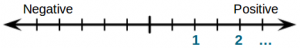Figure 1: Representation of the natural numbers on the line number

The mathematical symbol of the natural numbers is the capital letter N with double diagonal lines and an asterisk symbol:

$\huge \mathbb{N}^*$

## Whole Numbers

The whole numbers contain all natural (counting) numbers plus 0 (zero): 0, 1, 2, 3, …

As example, we can use whole numbers when we would like to express the amount of money we have:

• 0 $(no money), 10$, 100 $On the number line, the whole numbers contain also the 0 (zero) value: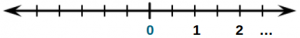Figure 2: Representation of the whole numbers on the line number The mathematical symbol for whole numbers is the capital letter N with double diagonal lines: $\huge \mathbb{N}$ ## Integer Numbers If we need to represent negative values, the whole numbers are not enough since they are only positive. Integer numbers contain all the whole numbers in both positive and negative form: …, -3, -2, -1, 0, 1, 2, 3, … We can use integer numbers to represent: • air temperature: -40 °C, 0 °C, +20 °C • financial situation: -1000$ (debt), +2000 \$ (profit)

On the number line the integer numbers cover also the left side, the negative numbers: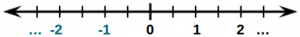Figure 3: Representation of the integer numbers on the line number

The mathematical symbol for integer numbers is the capital letter Z with double diagonal lines:

$\huge \mathbb{Z}$

## Rational Numbers

Rational numbers are the numbers that can be represented as a fraction of two integer numbers.

$\frac{x}{y}$

with the following properties:

$x \in \mathbb{Z}, y \in \mathbb{Z}, y\neq 0$

which mean that both x (numerator) and y (denominator) must be integers and also y non-zero.

Rational numbers can be represented as:

• fractions: -1/2, -3/2, -1/1, 1/3, 4/2, 7/2
• decimals: -0.5, -1.5, -1, 0.3333(3), 2, 3.5

Rational numbers contain all integer numbers because if y is 1 (one) we’ll have a division by 1 which gives an integer. Example: 4/1 = 4, -5/1 = -5

If we divide 1 to 3 we’ll get infinite decimal places. In this case the decimal representation is as follows: 1.3333(3) where 3 in round brackets means that it’s never ending.

Rational numbers can be used for example to describe slices (parts) of an item: 1/2 of an apple, 2/3 of a bread.

On the number line rational numbers are on both sides, negative and positive, and can be written as fractions or decimals: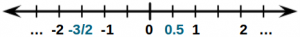Figure 4: Representation of the rational numbers on the line number

The mathematical symbol for rational numbers is the capital letter Q with double vertical lines:

$\huge \mathbb{Q}$

## Irrational Numbers

As the names suggests Irrational Numbers are numbers that are not rational, they can not be expressed as a ratio between two integers.

Irrational numbers are a special kind of numbers, most commonly used being:

• π (Greek letter pi): 3.1415926535897931159980…
• e (the base of the natural logarithm): 2.7182818284590450907956…

As you can see they have never ending decimal places and can not be written as the ratio between two integer numbers.

Another example of irrational numbers is the result of the square root function:

$\sqrt{x}, x \in \mathbb{N}$

If x is an natural number the square root of it will be a irrational number:

$\sqrt{2} = 1.4142135623730951454746…$

Irrational numbers are used all over the place in Mathematics, Physics, Economics, etc. An example of irrational number is the area of the circle with the radius of 1 meter:

$A_{circle}[m^2]=\pi r^2 = \pi = 3.14159…$

On the number line, irrational numbers can be found on both sides of zero (0), being negative or positive: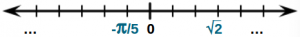Figure 5: Representation of the irrational numbers on the line number

The mathematical symbol for irrational numbers is the capital letter I with double vertical lines:

$\huge \mathbb{I}$

## Real Numbers

If we combine both the rational and the irrational numbers in a group of numbers we’ll get the real numbers. So the real numbers contains both real and irrational numbers.

Any place on the number line can be taken by a real number. Since they contain all rational numbers, they contain natural, whole and integer numbers as well as the irrational numbers.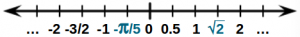Figure 6: Representation of the real numbers on the line number

The mathematical symbol for real numbers is the capital letter R with double vertical lines:

$\huge \mathbb{R}$

## Complex Numbers

Things are getting a bit more complicated with complex numbers.

Before diving into complex numbers let’s discuss a bit about the power of two function and the square root function.

If we take the number 2 and multiply it by itself we get 4. The same, if we take -2 and multiply it by itself we get also 4:

$2 \cdot 2 = 4$ $-2 \cdot -2 = 4$

This means that every number, positive or negative, multiplied by itself will produce all the time a positive number. Which means that the power of two function will only produce positive numbers:

$f(x) = x^{2} >= 0$

From this reasoning, we can say that the inverse function, the square root, makes sens only for positive numbers?

$f(x) = \sqrt{x}, \hspace{10mm} x \in \mathbb{N}$

If we want to know what is the square root of a negative number, we need to use complex numbers. In this case real numbers can not be used to describe the result.

Complex numbers are a special case of numbers which have the following properties:

• a complex number z has the general form of:
$z = a + b \cdot i$
• z has a real part (a) and an imaginary part (b)
• a and b are real numbers

Complex numbers are used in Mathematics, Physics and the fields of Engineering.

Examples of complex numbers: -3 – 2·i, 1 – 5·i, -3 + 3·i, -2 – 2·i

If the real part (a) of a complex number is 0, the complex number become purely imaginary. Also, if the imaginary part (b) is 0, the complex number becomes a real number.

Example of imaginary numbers: 2·i, -5·i

Complex numbers can not be represented anymore only on the number line (horizontal). They need two axis for representation:

• an horizontal axis for the real part
• a vertical axis for the imaginary part

The mathematical symbol for real numbers is the capital letter C with double vertical lines:

$\huge \mathbb{C}$

## Types of Numbers Overview

In Mathematics you’ll come across this expression:

$\Large \mathbb{N}^* \subset \mathbb{N} \subset \mathbb{Z} \subset \mathbb{Q} \subset \mathbb{R} \subset \mathbb{C}$

which says that complex complex numbers contain all real numbers, which contain all rational numbers and irrational numbers. Rational numbers contain all integer numbers, which contain all whole numbers, which contain all natural numbers.

In order to have a better understanding of all types of numbers you can save the picture below, which summarizes all the number types, examples and relationship between them.

Don’t forget to:

$\begin{equation*} \begin{split} \bbox[#FFFF9D]{f(x_i) = \left\{\begin{matrix} Subscribe,& i = 1\\Comment,& i = 2\\Like,& i = 3\\Share,& i = 4\\Support,& i = 5\end{matrix}\right.} \end{split} \end{equation*}$